## Perlin Noise

One of other common form of noise is perlin noise. Perlin noise is one implementation of so called “gradient noise” similarly to value noise it’s based on cells so it can be easily repeated and looks smooth. What differentiates it from value noise is that instead of interpolating the values, the values are based on inclinations. Because noise in general is a pretty complex topic I recommend you to read the tutorials on white noise and value noise first.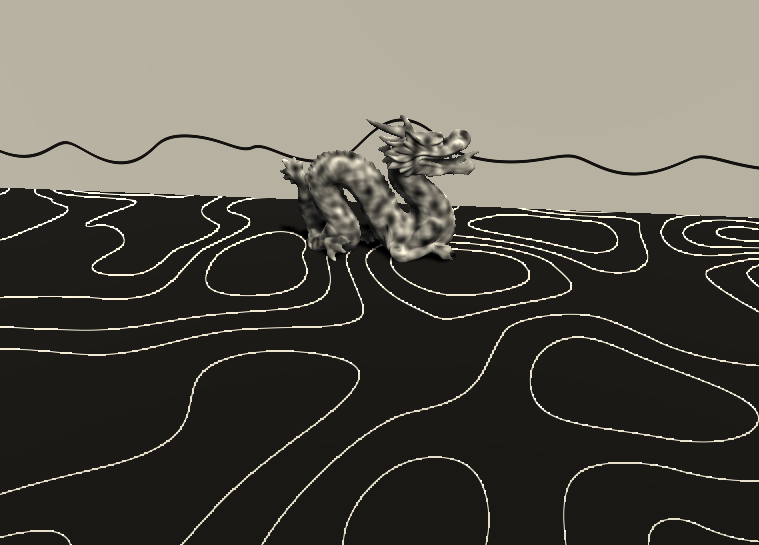## Gradient Noise in one Dimension

Perlin noise is a specific implementation of gradient noise for multiple dimensions. But generating gradient noise in one dimension is also pretty simple, so we’ll start with that.

This first implementation will, just like in the previous noise tutorials, be just in one dimension. We start with the 1d value noise shader. First we move the code of the noise function in it’s own function for more readability.

``````float gradientNoise(float value){
float previousCellNoise = rand1dTo1d(floor(value));
float nextCellNoise = rand1dTo1d(ceil(value));
float interpolator = frac(value);
interpolator = easeInOut(interpolator);
return lerp(previousCellNoise, nextCellNoise, interpolator);
}

void surf (Input i, inout SurfaceOutputStandard o) {
float value = i.worldPos.x / _CellSize;
float noise = perlinNoise(value);

float dist = abs(noise - i.worldPos.y);
float pixelHeight = fwidth(i.worldPos.y);
float lineIntensity = smoothstep(2*pixelHeight, pixelHeight, dist);
o.Albedo = lerp(1, 0, lineIntensity);
}
``````

Like mentioned previously, perlin noise doesn’t interpolate the values, it interpolates between directions. That means we start by generating a random inclination. This inclination can both go up and down, so we multiply our random value by 2 to move it to the 0 to 2 range and then subtract 1 to move it between -1 and +1.

After generating the inclination we get the value of the line with the chosen generated inclination based on the fractional part of our value. Because the typical equation of a line is `base + inclination * variable` and when we use the fractional part as a variable the line originates at 0 so our line equation is simply `inclination * fractional part`.

``````float gradientNoise(float value){
float fraction = frac(value);

float previousCellInclination = rand1dTo1d(floor(value)) * 2 - 1;
float previousCellLinePoint = previousCellInclination * fraction;

return previousCellLinePoint;
}
``````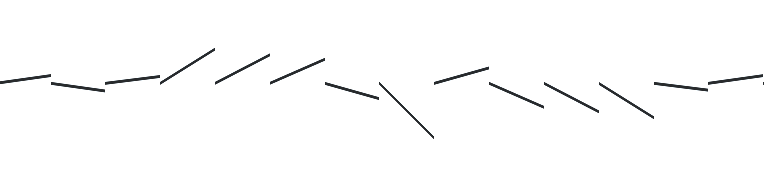For proper smoothing, we have to generate the line for the next cell too. The line is then on the left of the cell center, so we have to use negative values approaching zero for our variable. To get those values, we simply subtract 1 from our fractional part. That way when we generate values on the left of the segment we start with 0 - 1 which equals -1 and approach 1 - 1 which equals 0. Similar to previous noise generation, we can get the random inclination of the next cell with `floor(value)+1` or `ceil(value)`

``````float nextCellInclination = rand1dTo1d(ceil(value)) * 2 - 1;
float nextCellLinePoint = nextCellInclination * (fraction - 1);
``````

The next step is similar to what we did for easing the interpolation, we want the value of the line of the previous cell at the beginning of the segment and the line of the next segment at the end. So we simply interpolate between those values based on where on the segment we are. We’ll still use the easing, just to make it look smoother.

``````float gradientNoise(float value){
float fraction = frac(value);
float interpolator = easeInOut(fraction);

float previousCellInclination = rand1dTo1d(floor(value)) * 2 - 1;
float previousCellLinePoint = previousCellInclination * fraction;

float nextCellInclination = rand1dTo1d(ceil(value)) * 2 - 1;
float nextCellLinePoint = nextCellInclination * (fraction - 1);

return lerp(previousCellLinePoint, nextCellLinePoint, interpolator);
}
``````

Another small thing I’d like to change before calling the 1d gradient noise done is that right now our `rand1dTo1d` function always returns exactly 0 when we input zero, because of the calculations we do in it. What I’ll do for now to fix that is to change the mutator variable from a multiplication to a simple addition with a unusual number, so we don’t have that abnormal looking value at the origin. (Those changes are in the Random.cginc library file)(for every one looking at this later, I might have already changed that in the white noise tutorial💖)

``````float rand1dTo1d(float3 value, float mutator = 0.546){
float random = frac(sin(value + mutator) * 143758.5453);
return random;
}
``````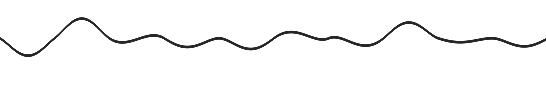## 2d Perlin Noise

For multidimensional perlin noise we can’t simply use a normal formula for a 1d line. Instead we interpolate the fraction in multiple dimensions and take the dot product with generated vectors of cells. To make the lines we generate with the dot product go to zero near the cell point itself, we scale the vector. That’s because of how the dot product works, a dot product with a `(0, 0)` vector will always be zero and a dot product with any vector and `(1, 0)` will always be twice as big as a dot product between `(0.5, 0)` and the same vector. Using the dot product this way means that we can use multiple dimensions as input well, but output will always be limited to one dimension.

``````float perlinNoise(float2 value){
float fraction = frac(value);
float interpolator = easeInOut(fraction);

float previousCellInclination = rand1dTo1d(floor(value)) * 2 - 1;
float previousCellLinePoint = previousCellInclination * fraction;

float nextCellInclination = rand1dTo1d(ceil(value)) * 2 - 1;
float nextCellLinePoint = nextCellInclination * (fraction - 1);

return lerp(previousCellLinePoint, nextCellLinePoint, interpolator);
}
``````

The first step of the implementation is generating 4 vectors in the 4 nearest cells, similarly to value noise. For that we can simply use the `rand2dTo2d` function we wrote in the white noise tutorial. Similarly to the 1d gradient noise, we want those vectors to point into all directions, not just to the top right in the 0 to 1 range like the random function returns. To fix that, we simply take the output of the random function, multiply it by 2 and subtract 1 again, the operations will automatically be applied to all components of the vector even though we only write down the scalar values.

``````float2 lowerLeftDirection = rand2dTo2d(float2(floor(value.x), floor(value.y))) * 2 - 1;
float2 lowerRightDirection = rand2dTo2d(float2(ceil(value.x), floor(value.y))) * 2 - 1;
float2 upperLeftDirection = rand2dTo2d(float2(floor(value.x), ceil(value.y))) * 2 - 1;
float2 upperRightDirection = rand2dTo2d(float2(ceil(value.x), ceil(value.y))) * 2 - 1;
``````

Then we generate the values again. They start at 0 at the cell, and then become bigger the further away they go.

For the lower left cell, which is equivalent to the previous cell in the 1d example, we can simply use the fraction as a vector as it is 0 at the cell and the y component becomes bigger the more we go up and the x component grows as we look further to the right, both increasing the absolute value of the result. On the lower right cell, we subtract `(1, 0)` from the value, so the vector will be smallest in the lower right corner and grow as we go left or up. Similarly to the 1d example we can also see here, that the value is negative when we approach the cell from the lower side, giving us continuous functions passing 0 at the cell position. And in the same matter we subtract `(0, 1)` from the fraction before taking the dot product with the upper left corner and subtract `(1, 1)` in case of the upper right corner.

``````float2 fraction = frac(value);

float2 lowerLeftFunctionValue = dot(lowerLeftDirection, fraction - float2(0, 0));
float2 lowerRightFunctionValue = dot(lowerRightDirection, fraction - float2(0, 1));
float2 upperLeftFunctionValue = dot(upperLeftDirection, fraction - float2(1, 0));
float2 upperRightFunctionValue = dot(upperRightDirection, fraction - float2(1, 1));
``````

Now that we generated all of our function values based on the random vectors we can interpolate between them like we’re used to. First the upper and lower pairs and then between the interpolated results.

``````float interpolatorX = easeInOut(fraction.x);
float interpolatorY = easeInOut(fraction.y);

float lowerCells = lerp(lowerLeftFunctionValue, lowerRightFunctionValue, interpolatorX);
float upperCells = lerp(upperLeftFunctionValue, upperRightFunctionValue, interpolatorX);

float noise = lerp(lowerCells, upperCells, interpolatorY);
return noise;
``````

Now that we have the whole noise function, we can now display it. Because the function fluctuates around 0 and approximately goes up and down by about 0.5, we’ll add 0.5 to the result to get noise approximately from 0 to 1.

``````void surf (Input i, inout SurfaceOutputStandard o) {
float2 value = i.worldPos.xz / _CellSize;
float noise = perlinNoise(value) + 0.5;

o.Albedo = noise;
}
``````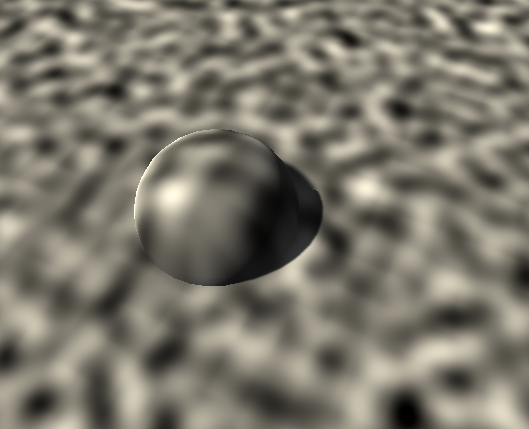## 3d Perlin Noise

For 3d we’ll implement the readable version with nested loops again. It looks very similar to the 3d value noise shader we wrote, but instead of just writing the random values to the values to interpolate in the innermost loop, we generate a random direction based on the cell. Then we also generate the comparison vector by subtracting the same value we used to get the cell from the fractional vector. After we have both of those vectors, we simply take the dot product between the two vectors and assign it to the noise value we interpolate. The rest of the function looks just like the 3d value noise function we wrote earlier.

``````float perlinNoise(float3 value){
float3 fraction = frac(value);

float interpolatorX = easeInOut(fraction.x);
float interpolatorY = easeInOut(fraction.y);
float interpolatorZ = easeInOut(fraction.z);

float3 cellNoiseZ;
[unroll]
for(int z=0;z<=1;z++){
float3 cellNoiseY;
[unroll]
for(int y=0;y<=1;y++){
float3 cellNoiseX;
[unroll]
for(int x=0;x<=1;x++){
float3 cell = floor(value) + float3(x, y, z);
float3 cellDirection = rand3dTo3d(cell) * 2 - 1;
float3 compareVector = fraction - float3(x, y, z);
cellNoiseX[x] = dot(cellDirection, compareVector);
}
cellNoiseY[y] = lerp(cellNoiseX, cellNoiseX, interpolatorX);
}
cellNoiseZ[z] = lerp(cellNoiseY, cellNoiseY, interpolatorY);
}
float3 noise = lerp(cellNoiseZ, cellNoiseZ, interpolatorZ);
return noise;
}
``````

For the input of the 3d noise, we now have to use 3d values as a input. With this 3d noise, we can then make coherent noise in 3d space without having to worry about generating 2d UVs or anything like that.

``````void surf (Input i, inout SurfaceOutputStandard o) {
float3 value = i.worldPos / _CellSize;
//get noise and adjust it to be ~0-1 range
float noise = perlinNoise(value) + 0.5;

o.Albedo = noise;
}
``````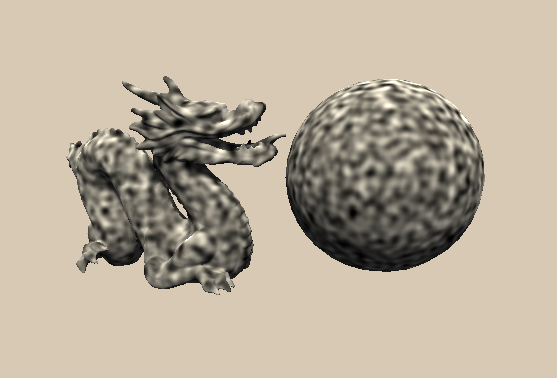## Special Use Case

Perlin noise itself usually just looks like weird clouds, but we can do some interresting effects with it if we know what we want.

As a first interresting thing, we can visualize lines where the noise has the same height, similar to height lines on maps. To archieve that we multiply the noise to make the noise span a wider range. Then we take the fractional amount of that value and display it.

``````float3 value = i.worldPos / _CellSize;
//get noise and adjust it to be ~0-1 range
float noise = perlinNoise(value) + 0.5;

noise = frac(noise * 6);

o.Albedo = noise;
``````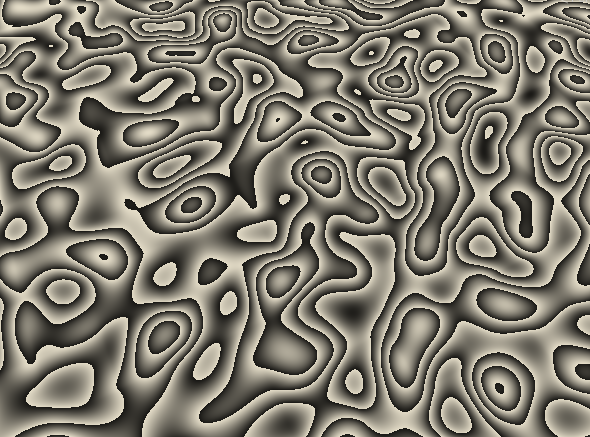Then we can then make smooth lines from that. First we have to find out how how much the noise changes in one pixel distance, for that we simply use the fwidth function. Then we can make a smooth half line at the top of the fractional range, so near 1, by using the smoothstep function.

We give the smoothstep function 1 minus the amount the noise changes in the neighboring pixels as the first parameter, one as the second parameter and the noise itself as the third parameter. That way the function will return 0 for all values that are more than 1 pixel away, and interpolate to a value of 1 until it reaches 1, which is the maximum value after we appied the frac function. Similarly we do a smoothstep for the lower end of the range. We feed it the change of the noise to the neighboring pixels as a first parameter, 0 as a second one and simply the fraction of the noise as the third parameter. This function will then return 0 for all values over the noise pixel change and then interpolate to 1 towards 0. To get the whole line, we’ll simply add the two values and return them.

``````void surf (Input i, inout SurfaceOutputStandard o) {
float3 value = i.worldPos / _CellSize;
//get noise and adjust it to be ~0-1 range
float noise = perlinNoise(value) + 0.5;

noise = frac(noise * 6);

float pixelNoiseChange = fwidth(noise);

float heightLine = smoothstep(1-pixelNoiseChange, 1, noise);
heightLine += smoothstep(pixelNoiseChange, 0, noise);

o.Albedo = heightLine;
}
``````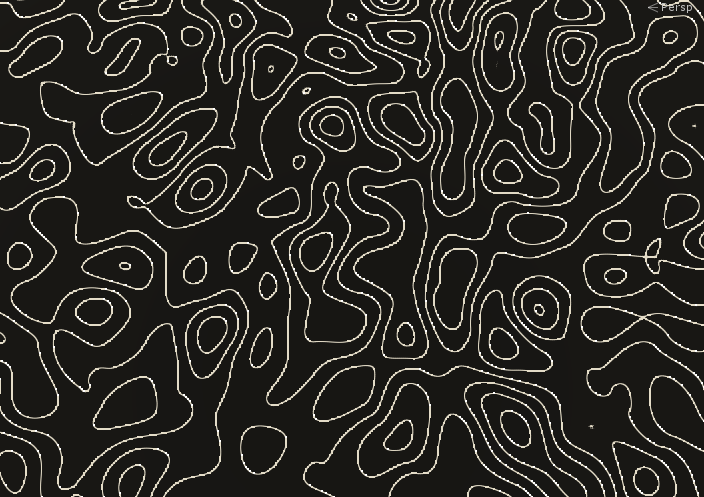And a last nice trick is to use the 3d noise function in situations where you’d only need the 2d function. That allows you to factor the time into the 3rd dimension and animate the noise without scrolling. If you made a 4d implementation of perlin noise you could also animate the 4th dimension to get a similar effect in 3 dimensions.

For that we simply add the time variable to the component we don’t need before we pass it to the noise function.

``````Properties {
_CellSize ("Cell Size", Range(0, 1)) = 1
_ScrollSpeed ("Scroll Speed", Range(0, 1)) = 1
}
``````
``````//global variables
float _CellSize;
float _ScrollSpeed;
``````
``````float3 value = i.worldPos / _CellSize;
value.y += _Time.y * _ScrollSpeed;
//get noise and adjust it to be ~0-1 range
float noise = perlinNoise(value) + 0.5;
``````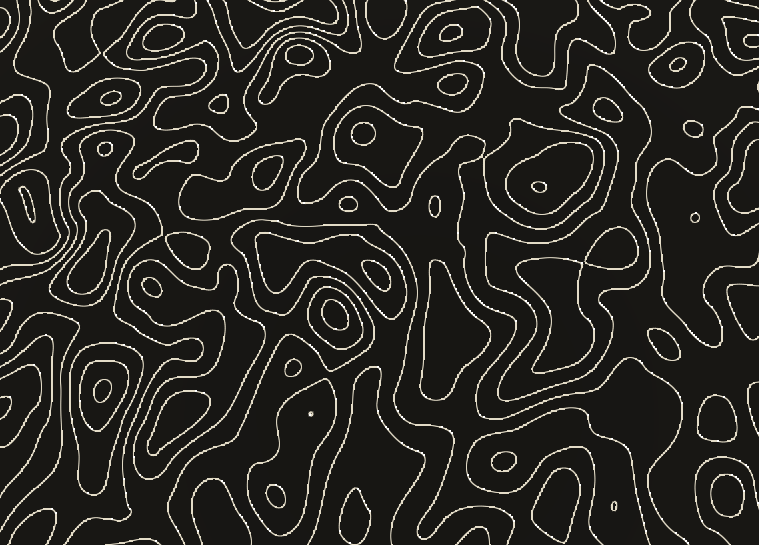## Source

``````Shader "Tutorial/026_perlin_noise/1d" {
Properties {
_CellSize ("Cell Size", Range(0, 1)) = 1
}
Tags{ "RenderType"="Opaque" "Queue"="Geometry"}

CGPROGRAM

#pragma target 3.0

#include "Random.cginc"

float _CellSize;

struct Input {
float3 worldPos;
};

float easeIn(float interpolator){
return interpolator * interpolator * interpolator * interpolator * interpolator;
}

float easeOut(float interpolator){
return 1 - easeIn(1 - interpolator);
}

float easeInOut(float interpolator){
float easeInValue = easeIn(interpolator);
float easeOutValue = easeOut(interpolator);
return lerp(easeInValue, easeOutValue, interpolator);
}

float fraction = frac(value);
float interpolator = easeInOut(fraction);

float previousCellInclination = rand1dTo1d(floor(value)) * 2 - 1;
float previousCellLinePoint = previousCellInclination * fraction;

float nextCellInclination = rand1dTo1d(ceil(value)) * 2 - 1;
float nextCellLinePoint = nextCellInclination * (fraction - 1);

return lerp(previousCellLinePoint, nextCellLinePoint, interpolator);
}

void surf (Input i, inout SurfaceOutputStandard o) {
float value = i.worldPos.x / _CellSize;

float dist = abs(noise - i.worldPos.y);
float pixelHeight = fwidth(i.worldPos.y);
float lineIntensity = smoothstep(2*pixelHeight, pixelHeight, dist);
o.Albedo = lerp(1, 0, lineIntensity);
}
ENDCG
}
FallBack "Standard"
}
``````

2d perlin noise

``````Shader "Tutorial/026_perlin_noise/2d" {
Properties {
_CellSize ("Cell Size", Range(0, 1)) = 1
}
Tags{ "RenderType"="Opaque" "Queue"="Geometry"}

CGPROGRAM

#pragma target 3.0

#include "Random.cginc"

float _CellSize;
float _Jitter;

struct Input {
float3 worldPos;
};

float easeIn(float interpolator){
return interpolator * interpolator;
}

float easeOut(float interpolator){
return 1 - easeIn(1 - interpolator);
}

float easeInOut(float interpolator){
float easeInValue = easeIn(interpolator);
float easeOutValue = easeOut(interpolator);
return lerp(easeInValue, easeOutValue, interpolator);
}

float perlinNoise(float2 value){
//generate random directions
float2 lowerLeftDirection = rand2dTo2d(float2(floor(value.x), floor(value.y))) * 2 - 1;
float2 lowerRightDirection = rand2dTo2d(float2(ceil(value.x), floor(value.y))) * 2 - 1;
float2 upperLeftDirection = rand2dTo2d(float2(floor(value.x), ceil(value.y))) * 2 - 1;
float2 upperRightDirection = rand2dTo2d(float2(ceil(value.x), ceil(value.y))) * 2 - 1;

float2 fraction = frac(value);

//get values of cells based on fraction and cell directions
float lowerLeftFunctionValue = dot(lowerLeftDirection, fraction - float2(0, 0));
float lowerRightFunctionValue = dot(lowerRightDirection, fraction - float2(1, 0));
float upperLeftFunctionValue = dot(upperLeftDirection, fraction - float2(0, 1));
float upperRightFunctionValue = dot(upperRightDirection, fraction - float2(1, 1));

float interpolatorX = easeInOut(fraction.x);
float interpolatorY = easeInOut(fraction.y);

//interpolate between values
float lowerCells = lerp(lowerLeftFunctionValue, lowerRightFunctionValue, interpolatorX);
float upperCells = lerp(upperLeftFunctionValue, upperRightFunctionValue, interpolatorX);

float noise = lerp(lowerCells, upperCells, interpolatorY);
return noise;
}

void surf (Input i, inout SurfaceOutputStandard o) {
float2 value = i.worldPos.xz / _CellSize;
//get noise and adjust it to be ~0-1 range
float noise = perlinNoise(value) + 0.5;

o.Albedo = noise;
}
ENDCG
}
FallBack "Standard"
}
``````

3d perlin noise

``````Shader "Tutorial/026_perlin_noise/3d" {
Properties {
_CellSize ("Cell Size", Range(0, 1)) = 1
}
Tags{ "RenderType"="Opaque" "Queue"="Geometry"}

CGPROGRAM

#pragma target 3.0

#include "Random.cginc"

float _CellSize;
float _Jitter;

struct Input {
float3 worldPos;
};

float easeIn(float interpolator){
return interpolator * interpolator;
}

float easeOut(float interpolator){
return 1 - easeIn(1 - interpolator);
}

float easeInOut(float interpolator){
float easeInValue = easeIn(interpolator);
float easeOutValue = easeOut(interpolator);
return lerp(easeInValue, easeOutValue, interpolator);
}

float perlinNoise(float3 value){
float3 fraction = frac(value);

float interpolatorX = easeInOut(fraction.x);
float interpolatorY = easeInOut(fraction.y);
float interpolatorZ = easeInOut(fraction.z);

float3 cellNoiseZ;
[unroll]
for(int z=0;z<=1;z++){
float3 cellNoiseY;
[unroll]
for(int y=0;y<=1;y++){
float3 cellNoiseX;
[unroll]
for(int x=0;x<=1;x++){
float3 cell = floor(value) + float3(x, y, z);
float3 cellDirection = rand3dTo3d(cell) * 2 - 1;
float3 compareVector = fraction - float3(x, y, z);
cellNoiseX[x] = dot(cellDirection, compareVector);
}
cellNoiseY[y] = lerp(cellNoiseX, cellNoiseX, interpolatorX);
}
cellNoiseZ[z] = lerp(cellNoiseY, cellNoiseY, interpolatorY);
}
float3 noise = lerp(cellNoiseZ, cellNoiseZ, interpolatorZ);
return noise;
}

void surf (Input i, inout SurfaceOutputStandard o) {
float3 value = i.worldPos / _CellSize;
//get noise and adjust it to be ~0-1 range
float noise = perlinNoise(value) + 0.5;

o.Albedo = noise;
}
ENDCG
}
FallBack "Standard"
}
``````

special use tricks

``````Shader "Tutorial/026_perlin_noise/special" {
Properties {
_CellSize ("Cell Size", Range(0, 1)) = 1
_ScrollSpeed ("Scroll Speed", Range(0, 1)) = 1
}
Tags{ "RenderType"="Opaque" "Queue"="Geometry"}

CGPROGRAM

#pragma target 3.0

#include "Random.cginc"

float _CellSize;
float _ScrollSpeed;

struct Input {
float3 worldPos;
};

float easeIn(float interpolator){
return interpolator * interpolator;
}

float easeOut(float interpolator){
return 1 - easeIn(1 - interpolator);
}

float easeInOut(float interpolator){
float easeInValue = easeIn(interpolator);
float easeOutValue = easeOut(interpolator);
return lerp(easeInValue, easeOutValue, interpolator);
}

float perlinNoise(float3 value){
float3 fraction = frac(value);

float interpolatorX = easeInOut(fraction.x);
float interpolatorY = easeInOut(fraction.y);
float interpolatorZ = easeInOut(fraction.z);

float3 cellNoiseZ;
[unroll]
for(int z=0;z<=1;z++){
float3 cellNoiseY;
[unroll]
for(int y=0;y<=1;y++){
float3 cellNoiseX;
[unroll]
for(int x=0;x<=1;x++){
float3 cell = floor(value) + float3(x, y, z);
float3 cellDirection = rand3dTo3d(cell) * 2 - 1;
float3 compareVector = fraction - float3(x, y, z);
cellNoiseX[x] = dot(cellDirection, compareVector);
}
cellNoiseY[y] = lerp(cellNoiseX, cellNoiseX, interpolatorX);
}
cellNoiseZ[z] = lerp(cellNoiseY, cellNoiseY, interpolatorY);
}
float3 noise = lerp(cellNoiseZ, cellNoiseZ, interpolatorZ);
return noise;
}

void surf (Input i, inout SurfaceOutputStandard o) {
float3 value = i.worldPos / _CellSize;
value.y += _Time.y * _ScrollSpeed;
//get noise and adjust it to be ~0-1 range
float noise = perlinNoise(value) + 0.5;

noise = frac(noise * 6);

float pixelNoiseChange = fwidth(noise);

float heightLine = smoothstep(1-pixelNoiseChange, 1, noise);
heightLine += smoothstep(pixelNoiseChange, 0, noise);

o.Albedo = heightLine;
}
ENDCG
}
FallBack "Standard"
}
``````

It took me a long time to understand how perlin noise works and I hope that by putting it into words here I made it easier for you, and that you’ll be able to create amazing effects with it.

You can also find the sources to the shaders of this tutorial here:

You can also find me on twitter at @totallyRonja. If you liked my tutorial and want to support me you can do that on Patreon (patreon.com/RonjaTutorials) or Ko-Fi (ko-fi.com/RonjaTutorials).